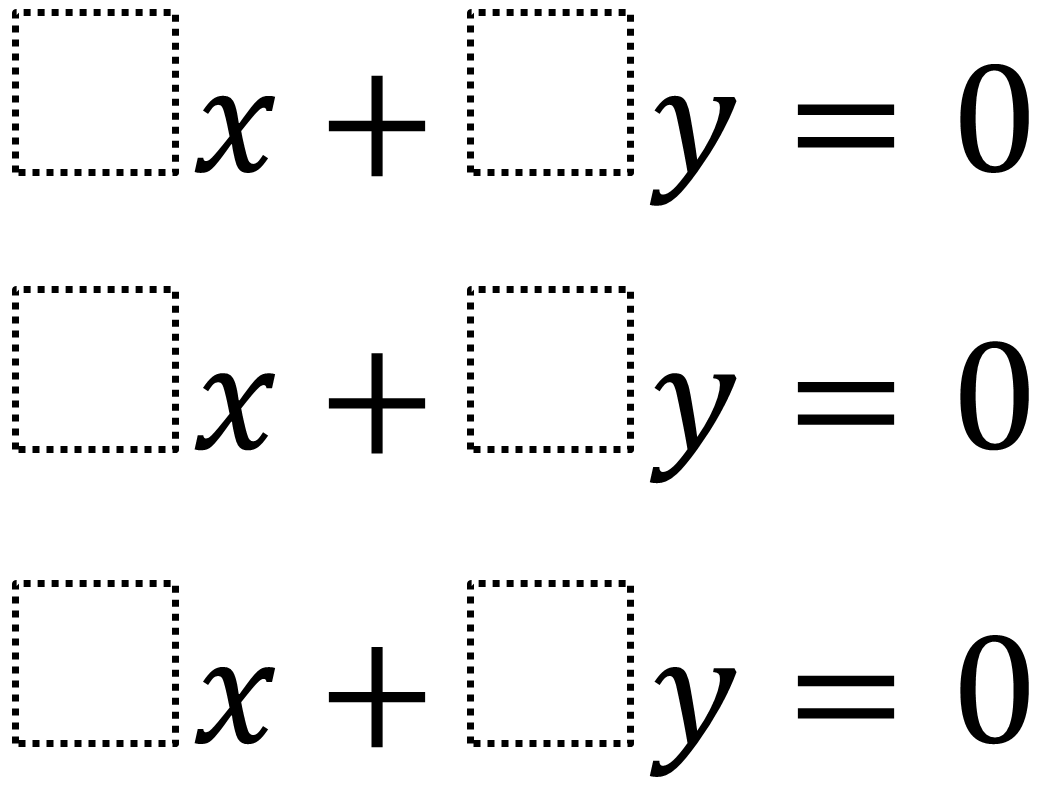# Systems of Three Equations – Multiple Solutions

Directions: Using the digits 1 through 9, at most one time each, create a system of equations that has as many solutions as possible.### Hint

How would three lines that have one solution look on a graph?  How would three lines that have two solutions look on a graph?  How would three lines that have three solutions look on a graph?  How would three lines that have more than 3 solutions look on a graph?